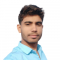## Realizing an AND Gate Using a 2:1 MUXUpdated on 19-Apr-2023 15:45:09
A multiplexer or MUX is a combinational circuit that accepts several data inputs and allows only one of them to flow through the output line. Multiplexer (MUX) is also known as data selector because it selects one from many. A MUX consists of 2n data input lines, n select lines, and 1 output line. Since, it converts 2n input lines into 1 output line. Therefore, it is also called many-to-one device. Depending upon the number of input lines, there are several types of multiplexer present such as 2:1 MUX, 4:1 MUX, 8:1 MUX, etc. Since, this article is meant for ... Read More

## Implementation of AND Gate from NOR GateUpdated on 02-Feb-2023 15:03:55
An AND Gate is a basic logic gate that performs the binary multiplication, i.e., it gives a HIGH or Logic 1 output, only when all its inputs are in HIGH or Logic 1 state. On the other hand, a NOR gate is a type of universal logic gate. Therefore, NOR gate can be used to realize any other type of logic gate. Before going into the implementation of AND gate using NOR gates only, let us discuss the basic theory of AND Gate and NOR Gate first. What is an AND Gate? An AND Gate is a basic logic gate. ... Read More

## Implementation of AND Gate from NAND GateUpdated on 02-Feb-2023 15:04:47
As we know that the NAND Gate is a universal logic gate, therefore using the NAND gate, we can implement any logic gate or any other logical expression. Read this tutorial to understand how you can implement an AND gate using NAND gate. Let's start with a basic overview of AND and NAND gates. What is an AND Gate? An AND Gate is a basic logic gate. An AND gate may have two or more than two inputs, but gives only one output. The AND gate gives a LOW (Logic 0) output if any one of its inputs is in ... Read More

## Implementation of a Logic Function using OR and AND GatesUpdated on 02-Feb-2023 15:02:57
We can realize or implement a Boolean expression or a logic function as hardware using logic gates. The easiest method of implementing a logic function as hardware using logic gates is to start with the output and moves towards the inputs. The implementation of a logic function with the help of logic gates involves connecting different logic gates in a specific manner. In this article, we shall confine our attention to implementing a logic function using the OR and AND gates only. Let's start the article with a brief introduction of OR and AND gates. What is an OR Gate? ... Read More